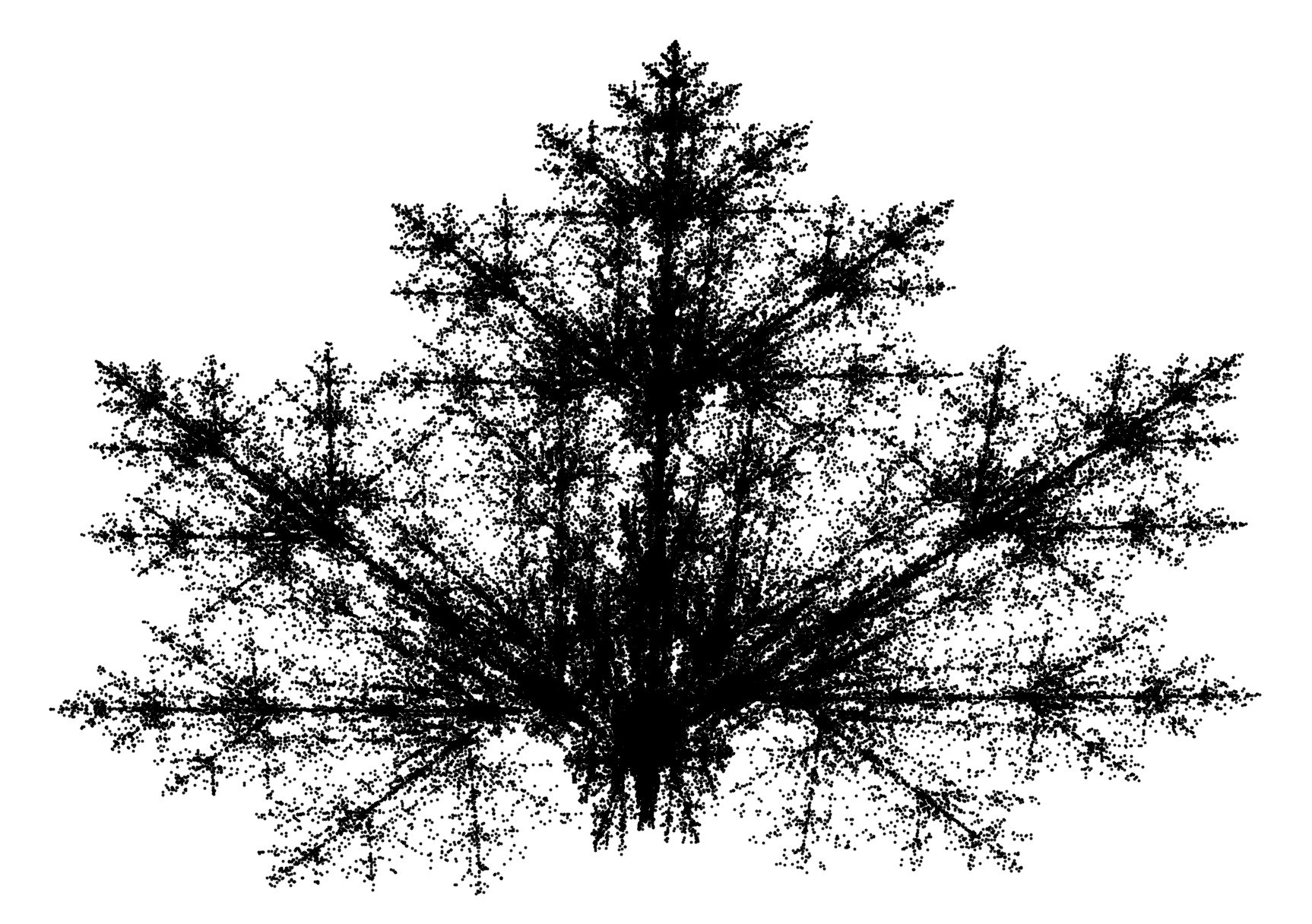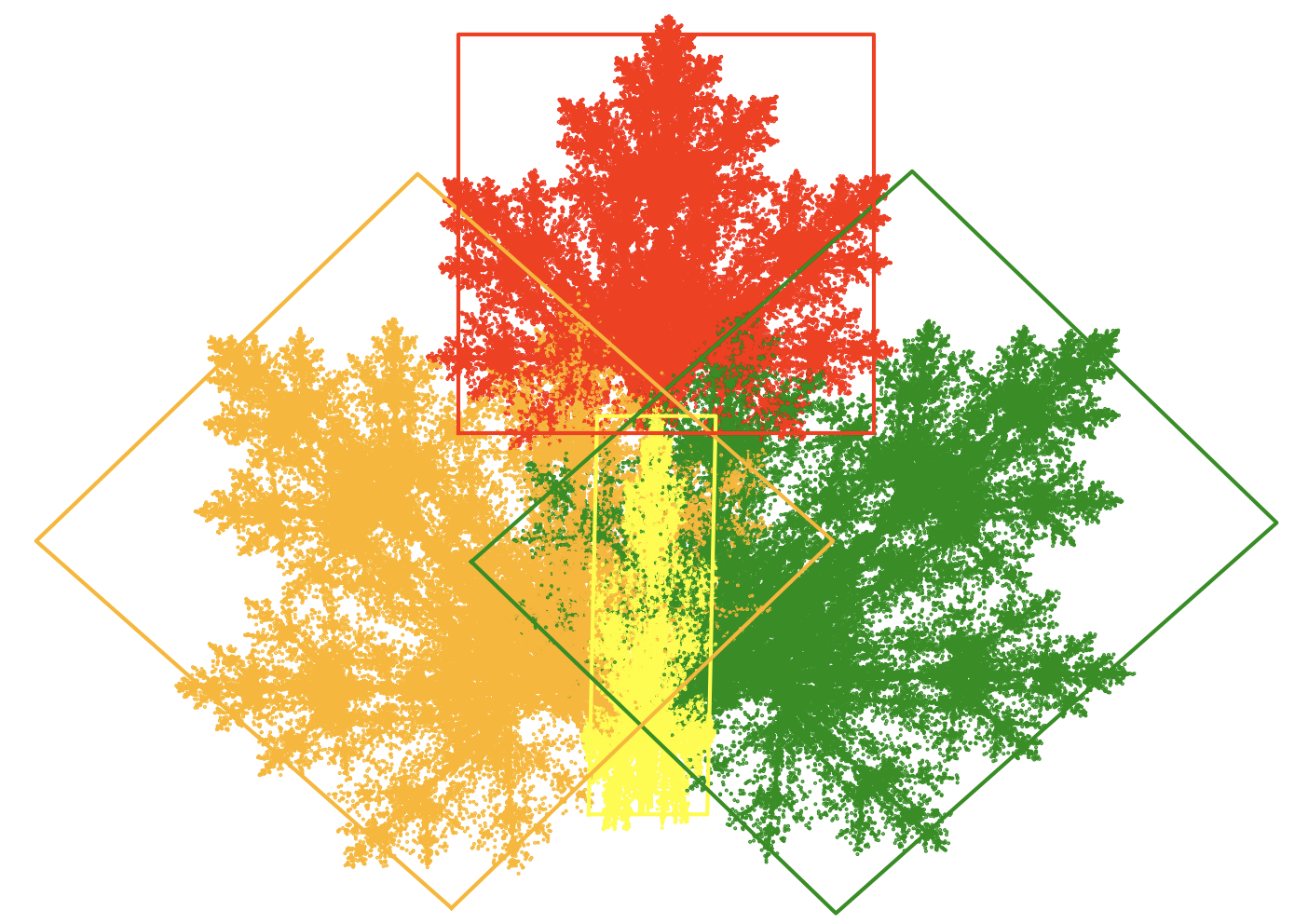## 4.2 Maple leafAn interesting fractal proposed by Paul Bourke. In construction it resembles a fern, but with different proportions of the individual components (smaller main leaf, larger side leaves and petiole). The image below illustrates a maple leaf and all four transformations.Transformations for the maple leaf. Color indicates the images resulting from successive transformations.

Graph obtained with the chaos game algorithm with probabilities $$\pi = [0.25; 0.25; 0.25; 0.25]$$.

$f_1(x, y) = \begin{bmatrix} 0.14 & 0.01 & -0.08 \\ 0 & 0.51 & -1.31 \end{bmatrix} [x \ y \ 1]^T$ $f_2(x, y) = \begin{bmatrix} 0.43 & 0.52 & 1.49 \\ -0.45 & 0.5 & -0.75 \end{bmatrix} [x \ y \ 1]^T$ $f_3(x, y) = \begin{bmatrix} 0.45 & -0.49 & -1.62 \\ 0.47 & 0.47 & -0.74 \end{bmatrix} [x \ y \ 1]^T$ $f_4(x, y) = \begin{bmatrix} 0.49 & 0 & 0.02 \\ 0 & 0.51 & 1.62 \end{bmatrix} [x \ y \ 1]^T$